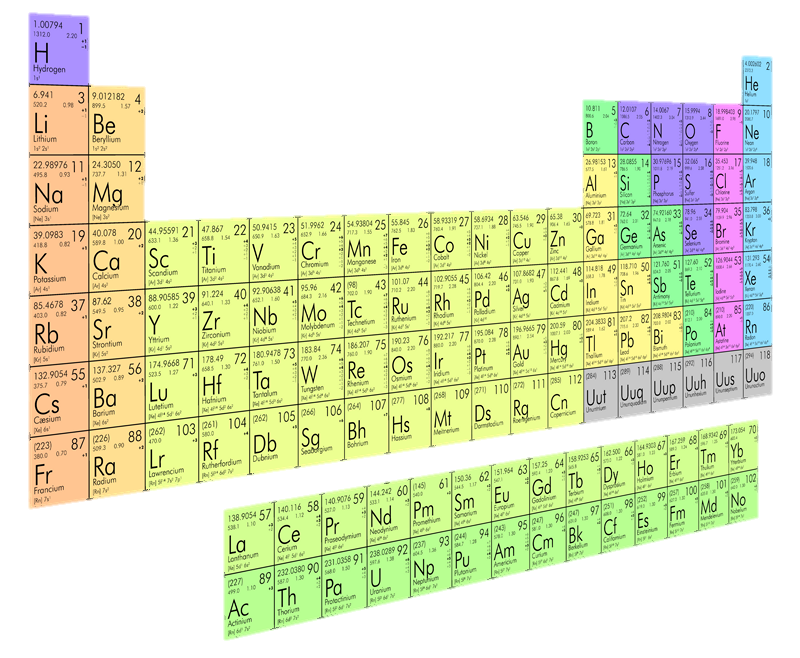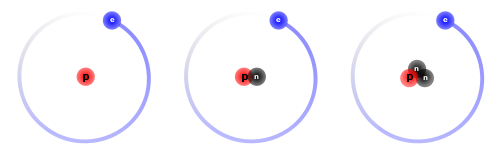# Elemental Words

 I wrote an article a few months ago about How much a body is worth? This earlier article described breaking the body into its constituent elements and estimating their value. In this article, I’m going to break a dictionary of words into its elemental components … sort of … I’m going to take a dictionary of words and find how many of these words can be written using only chemical element symbols. As you may know, each chemical element is given a distinct symbol of between one and three letters. The derivation of each symbol is rich in history and you can find many fascinating articles and books about this subject.For example the symbol for Mercury is  Hg   which stands for hydrargyrum or ‘liquid-silver’ in Greek.

Or Iron which is  Fe   and comes from the Latin ‘Ferrum’.

### Periodic Table

There are currently 118 identified chemical elementals. The last being Ununoctium  UuoEach element has its own distinct and unique symbol, with the exception of Hydrogen  HThere are three isotopes for Hydrogen – the other two being Deuterium (A Hydrogen atom with one additional Neutron), and Tritium (with two additional Neutrons). These two isotopes have the distinction of having their own elemental symbols. These are  D   and  T   respectively.

With these two additions it makes 120 different element symbols we can use. Because letters are used more than once in these symbols, often there are multiple ways to break apart a word into elements. For example the word PRINCESS can be broken down four different ways:

Pr   In   C   Es   S

Pr   In   Ce   S   S

Pr   I   N   C   Es   S

Pr   I   N   Ce   S   S

### Results

The dictionary file I used contained 172,806 distinct English words. Of these 47,035 (27.2%) could be written using element symbols.

The longest words found that can be made are: INTERSUBSTITUTABILITIES and NONREPRESENTATIONALISMS, both of which are 23 letters in length. Both of these can be represented multiple ways. Two examples for each word are shown below.

In   T   Er   S   U   B   S   Ti   T   U   Ta   B   I   Li   T   I   Es

I   N   T   Er   S   U   B   S   Ti   T   U   Ta   B   I   Li   T   I   Es

N   O   N   Re   P   Re   Se   N   T   At   I   O   Na   Li   Sm   S

No   N   Re   P   Re   Se   N   Ta   Ti   O   N   Al   I   Sm   S

Because element symbols can contain multiple letters, the longest English words do not necessarily make the longest elemental names. In fact the above words contain either 16 ot 17 elements, even though they are 23 letters long. The three words below each contain 19 elements, even though they are shorter.

P   S   Eu   D   O   S   O   P   H   I   S   T   I   Ca   T   I   O   N   S

I   N   T   Er   S   U   B   S   T   I   T   U   Ta   B   I   Li   T   I   Es

U   N   C   O   N   S   T   I   T   U   T   I   O   N   Al   I   T   I   Es

The top word can be made in three different ways with 19 elements (and 24 different ways in total). The other two just one way with 19 elements

The word NONANTIBIOTICS can be generated 32 different ways. The shortest contains just eight elements, whilst the longest contains thirteeen elements.

No   Na   N   Ti   Bi   O   Ti   Cs

N   O   Na   N   T   I   B   I   O   T   I   C   S

There are ten other words that have a difference of five between the shortest and longest representation (the largest spread observed in my database). These are: INCONCINNITIES, CONDITIONALITIES, CONDUCTIBILITIES, UNCONSTITUTIONALITIES, PHOTOCONDUCTIVITIES, INTERCONVERTIBILITIES, INCONVERTIBILITIES, CONSTITUTIONALITIES, POSTINOCULATION, BIOSTATISTICS.

The longest word found using all double letter elements is IRREPRESSIBILITIES (18 letters long).

Ir   Re   Pr   Es   Si   Bi   Li   Ti   Es

The longest word found using all single letter elements is PHOTOCONDUCTIVITY (17 letters long).

P   H   O   T   O   C   O   N   D   U   C   T   I   V   I   T   Y

### Highest Number of Canonical Forms

The word that can be spelled the most number of ways is POSTINOCULATION, of which there are 60 different variations of generation ranging from 9 to 14 elements (as noted above, this is one of the words with the greatest spread between the shortest and longest variations).

 P   Os   Ti   No   Cu   La   Ti   O   N Po   S   Ti   No   Cu   La   Ti   O   N Po   S   Ti   No   C   U   La   Ti   O   N Po   S   Ti   No   Cu   La   T   I   O   N Po   S   T   I   No   Cu   La   Ti   O   N Po   S   Ti   N   O   Cu   La   Ti   O   N P   Os   Ti   No   C   U   La   Ti   O   N P   Os   Ti   No   Cu   La   T   I   O   N P   Os   Ti   N   O   Cu   La   Ti   O   N Po   S   T   In   O   Cu   La   Ti   O   N P   O   S   Ti   No   Cu   La   Ti   O   N P   Os   T   In   O   Cu   La   Ti   O   N P   Os   T   I   No   Cu   La   Ti   O   N P   Os   T   I   No   C   U   La   Ti   O   N P   Os   T   I   No   Cu   La   T   I   O   N P   Os   T   I   N   O   Cu   La   Ti   O   N P   Os   T   In   O   C   U   La   Ti   O   N P   Os   T   In   O   Cu   La   T   I   O   N P   O   S   Ti   No   C   U   La   Ti   O   N P   O   S   Ti   No   Cu   La   T   I   O   N P   O   S   T   In   O   Cu   La   Ti   O   N P   O   S   T   I   No   Cu   La   Ti   O   N P   O   S   Ti   N   O   Cu   La   Ti   O   N Po   S   T   In   O   C   U   La   Ti   O   N Po   S   T   In   O   Cu   La   T   I   O   N Po   S   T   I   N   O   Cu   La   Ti   O   N P   Os   Ti   No   C   U   La   T   I   O   N P   Os   Ti   N   O   C   U   La   Ti   O   N P   Os   Ti   N   O   Cu   La   T   I   O   N Po   S   Ti   No   C   U   La   T   I   O   N Po   S   Ti   N   O   C   U   La   Ti   O   N Po   S   Ti   N   O   Cu   La   T   I   O   N Po   S   T   I   No   C   U   La   Ti   O   N Po   S   T   I   No   Cu   La   T   I   O   N Po   S   Ti   N   O   C   U   La   T   I   O   N Po   S   T   In   O   C   U   La   T   I   O   N Po   S   T   I   No   C   U   La   T   I   O   N Po   S   T   I   N   O   C   U   La   Ti   O   N Po   S   T   I   N   O   Cu   La   T   I   O   N P   O   S   Ti   No   C   U   La   T   I   O   N P   O   S   Ti   N   O   C   U   La   Ti   O   N P   O   S   Ti   N   O   Cu   La   T   I   O   N P   O   S   T   I   No   C   U   La   Ti   O   N P   O   S   T   I   No   Cu   La   T   I   O   N P   O   S   T   In   O   C   U   La   Ti   O   N P   O   S   T   In   O   Cu   La   T   I   O   N P   O   S   T   I   N   O   Cu   La   Ti   O   N P   Os   T   In   O   C   U   La   T   I   O   N P   Os   T   I   No   C   U   La   T   I   O   N P   Os   T   I   N   O   C   U   La   Ti   O   N P   Os   T   I   N   O   Cu   La   T   I   O   N P   Os   Ti   N   O   C   U   La   T   I   O   N P   Os   T   I   N   O   C   U   La   T   I   O   N P   O   S   T   I   No   C   U   La   T   I   O   N P   O   S   T   I   N   O   C   U   La   Ti   O   N P   O   S   T   I   N   O   Cu   La   T   I   O   N P   O   S   Ti   N   O   C   U   La   T   I   O   N Po   S   T   I   N   O   C   U   La   T   I   O   N P   O   S   T   In   O   C   U   La   T   I   O   N P   O   S   T   I   N   O   C   U   La   T   I   O   N

# Complete ListWant to see the complete list? Click here to see an alpha-sorted list of the words. (Opens in a new window).

You can find a complete list of all the articles here.Click here to receive email alerts on new articles.